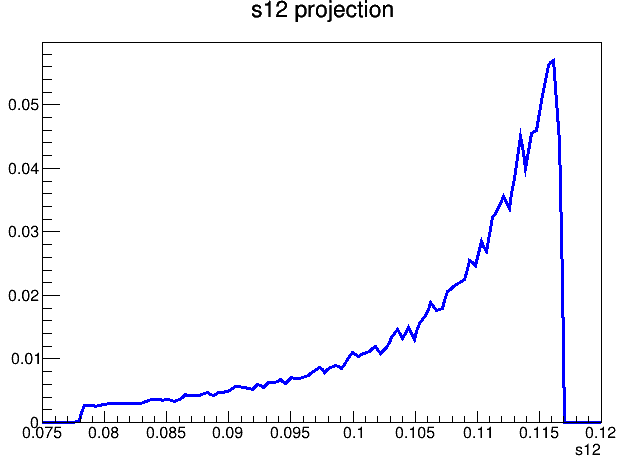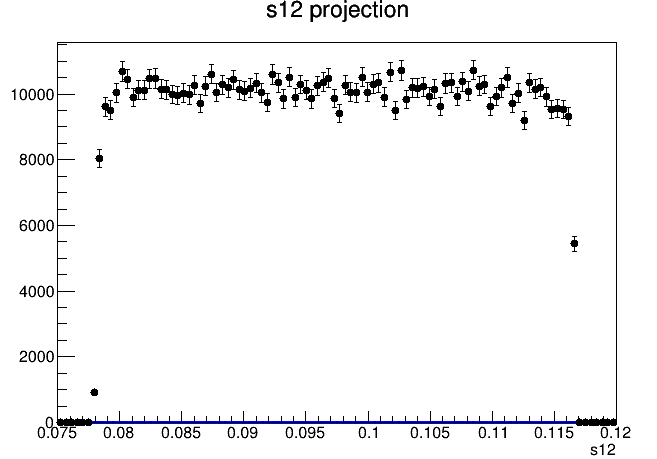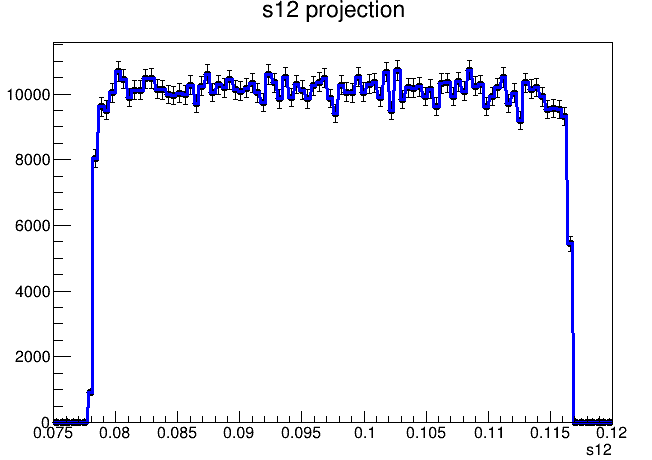# RooHistPdf plot depends on plot order

Hello experts,

I am trying to create a 2d pdf from a 2d weighted histogram, then use the 2d pdf to fit some data. The fit worked but there was something strange for the plotting.

1. If I plot `dataHistT1` only, I can get projection of the original histogram as expected.

2. If I plot `model` only, the plot seems to be normalized to 1 instead of the number of events. And the shape does not look right.3. If I plot `model` first, then `dataHistT1`. `model` seems to be normalized to 1.4. If I plot `dataHistT1` first, then `model`. I see a good agreement between the fitted model and the data.I am glad I found a way myself but I have no idea why 2) and 3) did not work. Can someone tell me what I did wrong?

Thanks

The code:

``````#include <iostream>
#include <vector>
#include <string>
#include <memory>

#include "TCanvas.h"
#include "TFile.h"
#include "TH2D.h"
#include "TFractionFitter.h"
#include "TObjArray.h"

#include "RooRealVar.h"
#include "RooDataHist.h"
#include "RooDataSet.h"
#include "RooHistPdf.h"
#include "RooHistFunc.h"
#include "RooRealSumPdf.h"
#include "RooPlot.h"
#include "RooGaussian.h"

using namespace RooFit;
using std::cout;
using std::endl;

void create_and_plot_pdf()
{

RooRealVar s12("s12", "s12", 0.075, 0.12);
RooRealVar s13("s13", "s13", 5.85, 6.2);

RooRealVar a1("a1", "T1 counts", 1e6, 0, 1e9);
auto fTemps = new TFile("amps.root");
auto histT1 = (TH2D*)fTemps->Get("hist_dalitz_T1");
auto dataHistT1 = new RooDataHist("dataHistT1", "dataHistT1",
RooArgSet(s12, s13), histT1);
// Create PDF from histograms with 1st order interpolation
RooHistPdf pdfT1("pdfT1", "pdfT1", RooArgSet(s12, s13), *dataHistT1, 1);

model.fitTo(*dataHistT1, Extended(kTRUE), Save());

RooPlot * s12Frame = s12.frame(Title("s12 projection"));

// pdfT1.plotOn(s12Frame);
dataHistT1->plotOn(s12Frame);
// Model has to be plotted at last to ensure correct normalization
model.plotOn(s12Frame);
s12Frame->Draw();
}
``````

This is expected, see here:

The short version of all this is:
Plot data first, because otherwise it’s not clear what the PDF should be normalised to. (In absence of any normalisation information, it’s normalised to unity).

Thanks for pointing that out! But one thing I do not understand is how can the normalization possibly change the shape of the PDF? The first plot looks drastically different from the shape of the data.

That could be the interpolation that’s applied. Did you try to compare with and without interpolation?

I tried to remove the interpolation but the shape stayed basically the same.

Could you check the coefficient of the AddPdf? Does the value of this coefficient change the shape?

Not sure if I understand what you mean but if I change the value of `a1`, the plot still looks like the first plot if I do not plot the data set first.

Ok, I don’t know why the shape changes, then.If you want to investigate further, I guess we need an example to run.

This topic was automatically closed 14 days after the last reply. New replies are no longer allowed.